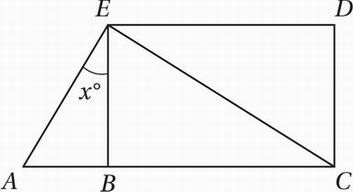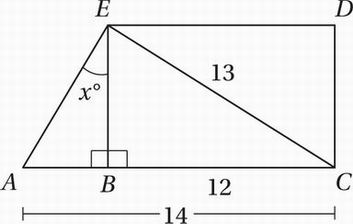# SAT Math Multiple Choice Question 727: Answer and Explanation

### Test Information

Question: 727

7.Note: Figure not drawn to scale.

In the figure above, BCDE is a rectangle, AC = 14, BC = 12, and EC = 13. What is the value of tan x?

• A. 0.4
• B. 0.6
• C. 1.3
• D. 2.5

Explanation:

AFirst, let's mark up the diagram with the given lengths, as above. Remember from SOH CAH TOA that the tangent of an angle is equal to the opposite side over the adjacent side, so tan x = AB/EB. AB = AC - BC = 14 - 12 = 2, and we can find EB with the Pythagorean Theorem:

EB2 + 122 = 132

Simplify:

EB2 + 144 = 169

Subtract 144:

EB2 = 25

Take the square root:

EB = 5

Or, even better, just notice that triangle EBC is a 5-12-13 triangle.

So, tan x = AB/EB = 2/5 = 0.4